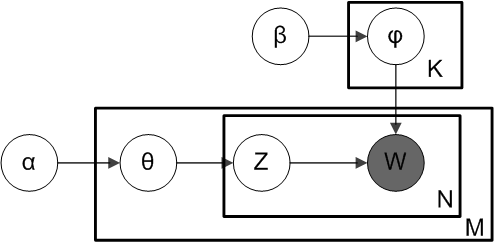LDA主题模型和Gibbs Sampling 学习整理

LDA主题模型模型我主要参考的是LDA数学八卦通俗理解LDA主题模型主题模型-LDA浅析进行学习和理解的。非常感谢他们的分享。

LDA模型

Dirichlet-Multinomial共轭

LDA必须要理解这个共轭结构，其实也没什么难的，就是下面的公式：

Dirichlet+Dirichlet

Dir(p|α)+Mult(n|p,N)=Dir(p|α+n)

LDA模型αθmzm,n$\overrightarrow \alpha \to \overrightarrow \theta_m \to z_{m,n}$

βφkωm,n|k=zm,n$\overrightarrow \beta \to \overrightarrow \varphi_k \to \omega_{m,n}|k=z_{m,n}$

θm=(θ(1)m,θ(2)m,,θ(k)m,,θ(K)m)

nm=(n(1)m,n(2)m,,n(k)m,,n(K)m)

θm=(θ(1)m,θ(2)m,,θ(k)m,,θ(K)m)

nm=(n(1)m,n(2)m,,n(k)m,,n(K)m)

Dir(θm|α)+Mult(nm|θm,Km)=Dir(θm|nm+α)

φk$\overrightarrow\varphi_k$是贝叶斯派所假设的变量，表示topic-doc骰子每个面出现的概率，一共存在V个面。φk$\overrightarrow\varphi_k$看成一个骰子，重复投掷Vk$V_k$次。φk$\overrightarrow\varphi_k$就是第k个topic的词分布
φk=(φ(1)k,φ(2)k,,φ(v)k,,φ(V)k)

nk=(n(1)k,n(2)k,,n(v)k,,n(V)k)

Dir(φk|β)+Mult(nk|φk,Vk)=Dir(φk|nk+β)

V代表取样次数，这里特指语料库词汇的总数
nk=(n(1)k,n(2)k,,n(t)k,,n(V)k)$\overrightarrow n_k=(n^{(1)}_k,n^{(2)}_k,\ldots,n^{(t)}_k,\ldots,n^{(V)}_k)$ 其中n(t)k$n^{(t)}_k$ 表示在V词取样中的词word=t的个数。

LDA公式推导

p(ω,z|α,β)$p(\overrightarrow \omega,\overrightarrow z | \overrightarrow \alpha, \overrightarrow \beta)$
(1)=p(ω|z,β)p(z|α)$(1)=p(\overrightarrow \omega | \overrightarrow z, \overrightarrow \beta )\cdot p(\overrightarrow z| \overrightarrow \alpha)$
(2)=Kk=1p(ωk|zk,β)Mm=1p(zm|α)$(2)=\prod_{k=1}^K p(\overrightarrow \omega_k| \overrightarrow z_k ,\overrightarrow \beta)\cdot\prod_{m=1}^Mp(\overrightarrow z_m | \overrightarrow \alpha)$
(3)=Kk=1p(ωk|β)Mm=1p(zm|α)$(3)=\prod_{k=1}^K p(\overrightarrow \omega_k | \overrightarrow \beta)\cdot\prod_{m=1}^M p(\overrightarrow z_m | \overrightarrow \alpha)$
(4)=Kk=1p(ωk|φk)p(φk|β)dφkMm=1p(zm|θm)p(θm|α)dθm$(4)=\prod_{k=1}^K \int p(\overrightarrow \omega_k | \overrightarrow \varphi_k)p(\overrightarrow \varphi_k | \overrightarrow \beta)d_{\overrightarrow \varphi_k} \cdot\prod_{m=1}^M \int p(\overrightarrow z_m | \overrightarrow \theta_m)p(\overrightarrow \theta_m | \overrightarrow \alpha)d_{\overrightarrow \theta_m}$
(5)=Kk=1Mult(nk|φk,Vk)Dir(φk|β)dφk$(5)=\prod_{k=1}^K\int Mult(\overrightarrow n_k|\overrightarrow\varphi_k,V_k)\cdot Dir(\overrightarrow\varphi_k|\overrightarrow\beta)d_{\overrightarrow\varphi_k}$
Mm=1Mult(nm|θm,Km)Dir(θm|α)dθm$\qquad\cdot\prod_{m=1}^M\int Mult(\overrightarrow n_m|\overrightarrow\theta_m,K_m)\cdot Dir(\overrightarrow\theta_m|\overrightarrow\alpha)d_{\overrightarrow\theta_m}$
(6)=Kk=1Vki=1φiknik1Δ(β)Vki=1φikβi1dφkMm=1Kmi=1θimnim1Δ(α)Kmi=1θimαi1dθk$(6)=\prod_{k=1}^K \int \prod_{i=1}^{V_k} {\varphi_k^i}^{n_k^i} \frac1{\Delta(\overrightarrow \beta)} \prod_{i=1}^{V_k} {\varphi_k^i}^{\beta_i -1}d_{\overrightarrow \varphi_k} \cdot \prod_{m=1}^M \int \prod_{i=1}^{K_m}{\theta_m^i}^{n_m^i}\frac1{\Delta(\overrightarrow\alpha)}\prod_{i=1}^{K_m}{\theta_m^i}^{\alpha_i -1}d_{\overrightarrow\theta_k}$
(7)=Kk=11Δ(β)Vki=1φiknik+βi1dφkMm=11Δ(α)Kmi=1θimnim+αi1dθm$(7)=\prod_{k=1}^K\frac1{\Delta(\overrightarrow\beta)}\int\prod_{i=1}^{V_k}{\varphi_k^i}^{n_k^i + \beta_i -1}d_{\overrightarrow\varphi_k}\cdot\prod_{m=1}^M\frac1{\Delta(\overrightarrow\alpha)}\prod_{i=1}^{K_m}{\theta_m^i}^{n_m^i + \alpha_i - 1}d_{\overrightarrow\theta_m}$
(8)=Kk=1Δ(nk+β)Δ(β)Mm=1Δ(nm+α)Δ(α)$(8)=\prod_{k=1}^K{{\Delta(\overrightarrow n_k + \overrightarrow\beta)}\over{\Delta(\overrightarrow\beta)}}\prod_{m=1}^M{{\Delta(\overrightarrow n_m + \overrightarrow\alpha)}\over{\Delta(\overrightarrow\alpha)}}$

Mult(nm|θm,Km)=i=1Kmθimnim

Dir(θm|α)=1Δ(α)Kmi=1θimαi1$Dir(\overrightarrow{\theta_m}|\overrightarrow\alpha)=\frac1{\Delta(\overrightarrow \alpha)}\prod_{i=1}^{K_m}{\theta_m^i}^{\alpha_i - 1}$，其中
Δ(α)=i=1Kmφimαi1dφm

Mult(nk|φk,Vk)=i=1Vkφikβi1

Dir(φk|β)=1Δ(β)Vmi=1nikβi1$Dir(\overrightarrow{\varphi_k}|\overrightarrow\beta)=\frac1{\Delta(\overrightarrow\beta)}\prod_{i=1}^{V_m}{{n_k^i}^{\beta_i -1}}$,其中
Δ(β)=i=1Vkθimβi1dθm

p(ωk|zk,β)p(ωk|zk,β)

LDA总结

p(ω,z|α,β)=p(ω|z,β)p(z|α)

=k=1KΔ(nk+β)Δ(β)m=1MΔ(nm+α)Δ(α)

Gibbs Sampling

p(zi=k|z¬i,ω)p(zi=k,ωi=t|z¬i,ω¬i)

αθmzm

βφkωk

p(θm|z¬i,ω¬i)=Dir(nm,¬i+α)

p(φk|z¬i,ω¬i)=Dir(nk,¬i+β)

Gibbs Sampling 公式推算

p(zi=k|z¬i,ω)p(zi=k,ωi=t|z¬i,ω¬i)$\quad p(z_i=k|\overrightarrow z_{\lnot i},\overrightarrow \omega)\propto p(z_i=k,\omega_i=t|\overrightarrow z_{\lnot i},\overrightarrow\omega_{\lnot i})$
=p(zi=k,ωi=t,θm,φ|z¬i,ω¬i)dθmdφk$=\int p(z_i = k ,\omega_i=t,\overrightarrow\theta_m,\overrightarrow\varphi|\overrightarrow z_{\lnot i},\overrightarrow\omega_{\lnot i})d_{\overrightarrow\theta_m}d_{\overrightarrow\varphi_k}$
=p(zi=k,θm|z¬i,ω¬i)p(ωi=t,φk|z¬i,ω¬i)dθmdφk$=\int p(z_i=k,\overrightarrow\theta_m|\overrightarrow z_{\lnot i},\overrightarrow\omega_{\lnot i})\cdot p(\omega_i=t,\overrightarrow\varphi_k|\overrightarrow z_{\lnot i},\overrightarrow\omega_{\lnot i})d_{\overrightarrow\theta_m}d_{\overrightarrow\varphi_k}$
=p(zi=k|θm)p(θm|z¬i,ω¬i)p(ωi=t|φk)p(φk|z¬i,ω¬i)dθmdφk$=\int p(z_i=k|\overrightarrow\theta_m)p(\overrightarrow\theta_m|\overrightarrow z_{\lnot i},\overrightarrow\omega_{\lnot i})\cdot p(\omega_i=t|\overrightarrow\varphi_k)p(\overrightarrow\varphi_k|\overrightarrow z_{\lnot i},\overrightarrow\omega_{\lnot i})d_{\overrightarrow\theta_m}d_{\overrightarrow\varphi_k}$
=p(zi=k|θm)Dir(θm|nm,¬i+α)dθmp(ωi=t|φ)Dir(φ|nk,¬i)dφk$=\int p(z_i=k|\overrightarrow\theta_m)Dir(\overrightarrow\theta_m|\overrightarrow n_{m,\lnot i}+\overrightarrow\alpha)d_{\overrightarrow\theta_m}\cdot\int p(\omega_i=t|\overrightarrow\varphi)Dir(\overrightarrow\varphi|\overrightarrow n_{k,\lnot i})d_{\overrightarrow\varphi_k}$
=θm,kDir(θm|nm,¬i+α)dθmφk,tDir(φ|nk,¬i)dφk$=\int \theta_{m,k}Dir(\overrightarrow\theta_m|\overrightarrow n_{m,\lnot i}+\overrightarrow\alpha)d_{\overrightarrow\theta_m}\cdot\int\varphi_{k,t}Dir(\overrightarrow\varphi|\overrightarrow n_{k,\lnot i})d_{\overrightarrow\varphi_k}$
=E(θm,k)E(φ(k,t)$=E(\theta_{m,k})\cdot E(\varphi_(k,t)$
=θ^m,kφ^k,t$=\hat\theta_{m,k}\cdot\hat\varphi_{k,t}$

Dirichlet参数估计公式，详见通俗理解LDA

θ^m,k=nkm,¬i+αkKk=1(nkm,¬i+αk)

φ^k,t=ntk,¬i+βtVt=1(ntk,¬i+βt)

p(zi=k|z¬i,ω)nkm,¬i+αkKk=1(nkm,¬i+αk)ntk,¬i+βtVt=1(ntk,¬i+βt)